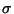Next:  The .mtz wrapper Up:  Using the program Previous:  Testing the executable   Contents

## Supported crystallographic calculations

GraphEnt automatically recognises the following types of syntheses :

• F2 Patterson synthesis, defined by h, k, l, F,(F)
•F2 difference Patterson synthesis, defined by h, k, l, F1,(F1), F2,(F2). The function calculated is the one commonly used for isomorphous difference Patterson maps with an amplitude of F = (F1 - F2)2 and a standard deviation(F) = 2+(F1)2 +(F2)2.
• Phased Fourier synthesis without FOM, defined by h, k, l, F,(F),• Phased Fourier synthesis with FOM, defined by h, k, l, F,(F),, FOM
All other types of syntheses that can be reduced to any of the above are also supported but the reduction step is a user's responsibility. For example, to calculate a (2Fo - Fc)exp(i) map you would have to prepare a column containing the (2Fo - Fc) term by yourself, and give the program six columns of the type h, k, l,(2Fo - Fc),(2Fo - Fc),. For CCP4 users this is easily (and interactively) achieved with the program sftools9.

#### Footnotes

... sftools9
I should add here that for some of the more complex syntheses, the most difficult part of setting-up the calculation appears to be the propagation of errors, ie calculating correctly the standard deviation of the amplitude terms.Next:  The .mtz wrapper Up:  Using the program Previous:  Testing the executable   Contents
NMG, Nov 2002